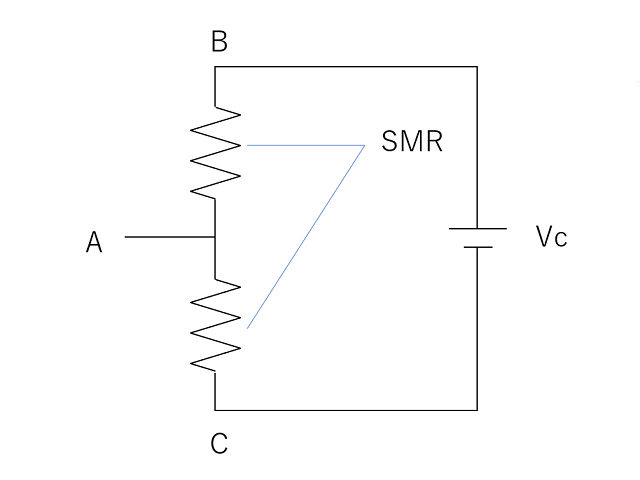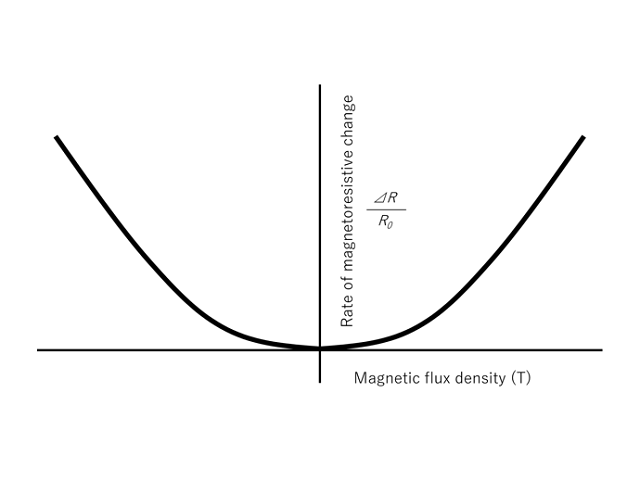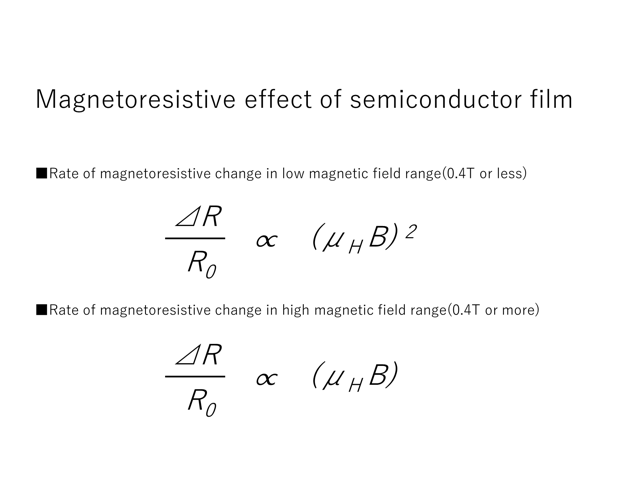FAQ & Glossary

Basic Knowledge of Magnetic Sensor

Hall elements and semiconductor magnetoresistive elements are the same in that they utilize the effect of the Lorentz force acting on carriers. The Hall element is a device that uses the Hall effect to detect the electromotive force generated by the Lorentz force as a voltage, and is used as a stand-alone element.

On the other hand, semiconductor magnetoresistive devices utilize the magnetoresistive effect to extract the change in the resistance value caused by the Lorentz force as a voltage. The actual device is configured with multiple resistive elements (two or four) in one package, each of which comprises many resistors connected in series. The voltage is divided by the resistance of these two or four elements and the result is extracted. With such a feature as this, semiconductor magnetoresistive devices are used to apply different magnetitic fields to 2 elements and 4 elements.

As described above, Hall elements and semiconductor magnetoresistive elements are not the same.. The differences between them can be better understood by examining their characteristics as sensors and their specific applications as described below.Configuration of semiconductor magnetoresistive element

Hall Elements

Since the electromotive force generated by the Lorentz force has a +/- polarity depending on the polarity of the magnetic field, Hall elements can detect the strength and direction of the magnetic field (S-pole/N-pole). They can also detect magnetic fields over a wide range of 0 to ±100 mT and are therefore used in applications where magnetic pole changes in this field range are to be detected.

Typical applications of Hall elements:
Detection of the S-pole and N-pole of a DC brushless motor, detection of the cycle of the S-pole and N-pole of a magnet to determine the number of rotations, etc.

Semiconductor Magnetoresistive Elements

Since semiconductor magnetoresistive elements use the change in resistance value generated by the Lorentz force, they can detect the strength of the magnetic field, but not the direction of the magnetic field (S-pole/N-pole).

Since the change in resistance of semiconductor magnetoresistive elements is very small in low magnetic fields (approximately 0 to several hundred mT), they are used to detect changes in magnetic fields in high magnetic fields (approximately 400 mT or more).

Another advantage of using them in a high magnetic field is that the linearity of the resistance change to the magnetic field is improved so that they are able to obtain an output waveform with less distortion in response to changes in the magnetic field. In actual use, it is common to install a bias magnet to increase the magnetic field.

Typical application examples of semiconductor magnetoresistive elements:
Detection of gear rotation, magnetic ink reading of banknotes, etc.Graph of relationship between M.F.D and rate of magnetoresistive changeMagnetoresistive effect of semiconductor film

Glossary

Here are some technology key words that often appear when you use sensors.

Magnetic field strength (H) multiplied by magnetic permeability (μ) is called magnetic flux density (B). The unit of magnetic flux density is generally Tesla [T].

In the case of a sensor with digital output, it refers to the minimum value of the magnetic field change required to change the output by one code. For example, in the case of a sensor whose sensitivity is "1.1 [μT / LSB]", when a magnetic field change of 1.1 [μT] occurs, the sensor's output code changes for the first time. This is synonymous with the resolution.

A tri-axis magnetic sensor is a sensor that can detect a magnetic field from any direction (X, Y, Z axes) applied to the sensor. In AKM, it is a group of products called "3-axis Magnetic Sensor" and "3D Magnetic Sensor."